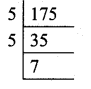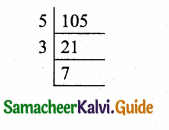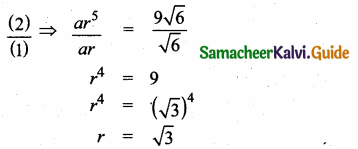Students can download Maths Chapter 2 Relations and Functions Unit Exercise 2 Questions and Answers, Notes, Samacheer Kalvi 10th Maths Guide Pdf helps you to revise the complete Tamilnadu State Board New Syllabus, helps students complete homework assignments and to score high marks in board exams.

## Tamilnadu Samacheer Kalvi 10th Maths Solutions Chapter 2 Numbers and Sequences Unit Exercise 2

Question 1.
Prove that n2 – n divisible by 2 for every positive integer n.
We know that any positive integer is of the form 2q or 2q + 1 for some integer q.
Case 1: When n = 2 q
n2 – n = (2q)2 – 2q = 4q2 – 2q
= 2q (2q – 1)
In n2 – n = 2r
2r = 2q(2q – 1)
r = q(2q + 1)
n2 – n is divisible by 2

Case 2: When n = 2q + 1
n2 – n = (2q + 1)2 – (2q + 1)
= 4q2 + 1 + 4q – 2q – 1 = 4q2 + 2q
= 2q (2q + 1)
If n2 – n = 2r
r = q (2q + 1)
∴ n2 – n is divisible by 2 for every positive integer “n”Question 2.
A milk man has 175 litres of cow’s milk and 105 litres of buffalow’s milk. He wishes to sell the milk by filling the two types of milk in cans of equal capacity. Calculate the following
(i) Capacity of a can
(ii) Number of cans of cow’s milk
(iii) Number of cans of buffalow’s milk.
175 litres of cow’s milk.
105 litres of goat’s milk.
H.C.F of 175 & 105 by using Euclid’s division algorithm.
175 = 105 × 1 + 70, the remainder 70 ≠ 0Again using division algorithm,105 = 70 × 1 + 35, the remainder 35 ≠ 0
Again using division algorithm.
70 = 35 × 2 + 0, the remainder is 0.
∴ 35 is the H.C.F of 175 & 105.
(i) ∴ The milk man’s milk can’s capacity is 35 litres.
(ii) No. of cow’s milk obtained = $$\frac { 175 }{ 35 }$$ = 5 cans
(iii) No. of buffalow’s milk obtained = $$\frac { 105 }{ 35 }$$ = 3 cansQuestion 3.
When the positive integers a, b and c are divided by 13 the respective remainders are 9,7 and 10. Find the remainder when a + 2b + 3c is divided by 13.
Given the positive integer are a, b and c
a = 13q + 9 (divided by 13 leaves remainder 9)
b = 13q + 7
c = 13q + 10
a + 2b + 3c = 13q + 9 + 2(13q + 7) + 3 (13q + 10)
= 13q + 9 + 26q + 14 + 39q + 30
= 78q + 53
When compare with a = 3q + r
= (13 × 6) q + 53
The remainder is 53

Question 4.
Show that 107 is of the form 4q +3 for any integer q.
Solution:
107 = 4 × 26 + 3. This is of the form a = bq + r.
Hence it is proved.Question 5.
If (m + 1)th term of an A.P. is twice the (n + 1)th term, then prove that (3m + 1)th term is twice the (m + n + 1)th term.
tn = a + (n – 1)d
Given tm+1 = 2 tn+1
a + (m + 1 – 1)d = 2[a + (n + 1 – 1)d]
a + md = 2(a + nd) ⇒ a + md =2a + 2nd
md – 2nd = a
d(m – 2n) = a ….(1)
To Prove t(3m + 1) = 2(tm+n+1)
L.H.S. = t3m+1
= a + (3m + 1 – 1)d
= a + 3md
= d(m – 2n) + 3md (from 1)
= md – 2nd + 3md
= 4md – 2nd
= 2d (2m – n)
R.H.S. = 2(tm+n+1)
= 2 [a + (m + n + 1 – 1) d]
= 2 [a + (m + n)d]
= 2 [d (m – 2n) + md + nd)] (from 1)
= 2 [dm – 2nd + md + nd]
= 2 [2 md – nd]
= 2d (2m – n)
R.H.S = L.H.S
∴ t(3m+1) = 2 t(m+n+1)
Hence it is proved.Question 6.
Find the 12th term from the last term of the A.P -2, -4, -6,… -100.
The given A.P is -2, -4, -6, …. 100
d = -4 – (-2) = -4 + 2 = – 2
Finding the 12 term from the last term
a = -100, d = 2 (taking from the last term)
n = 12
tn = a + (n – 1)d
t12 = – 100 + 11 (2)
= -100 + 22
= -78
∴ The 12th term of the A.P from the last term is – 78

Question 7.
Two A.P’s have the same common difference. The first term of one A.P is 2 and that of the other is 7. Show that the difference between their 10th terms is the same as the difference between their 21st terms, which is the same as the difference between any two corresponding terms.
Solution:
Let the two A.Ps be
AP1 = a1, a1 + d, a1 + 2d,…
AP2 = a2, a2 + d, a2 + 2d,…
In AP1 we have a1 = 2
In AP2 we have a2 = 7
t10 in AP1 = a1 + 9d = 2 + 9d ………….. (1)
t10 in AP2 = a2 + 9d = 7 + 9d …………… (2)
The difference between their 10th terms
= (1) – (2) = 2 + 9d – 7 – 9d
= -5 ………….. (I)
t21 m AP1 = a1 + 20d = 2 + 20d …………. (3)
t21 in AP2 = a2 + 20d = 7 + 20d ………… (4)
The difference between their 21 st terms is
(3) – (4)
= 2 + 20d – 7 – 20d
= -5 ……………. (II)
I = II
Hence it is Proved.Question 8.
A man saved ₹16500 in ten years. In each year after the first he saved ₹100 more than he did in the preceding year. How much did he save in the first year?
Amount of saving in ten years = ₹ 16500
S10 = 16500, d= 100
Sn = $$\frac { n }{ 2 }$$ [2a + (n – 1)d]
S10 = $$\frac { 10 }{ 2 }$$ [2a + 9d]
16500 = $$\frac { 10 }{ 2 }$$ [2a + 900] = 5(2a + 900)
16500 = 10a + 4500 ⇒ 16500 – 4500 = 10a
12000 = 10a
a = $$\frac { 12000 }{ 10 }$$ = 1200
Amount saved in the first year = ₹ 1200

Question 9.
Find the G.P. in which the 2nd term is $$\sqrt { 6 }$$ and the 6th term is 9 $$\sqrt { 6 }$$.
2nd term of the G.P = $$\sqrt { 6 }$$
t2 = $$\sqrt { 6 }$$
[tn = a rn-1]
a.r = $$\sqrt { 6 }$$ ….(1)
6th term of the G.P. = 9 $$\sqrt { 6 }$$
a. r5 = 9$$\sqrt { 6 }$$ ……..(2)Question 10.
The value of a motorcycle depreciates at a rate of 15% per year. What will be the value of the motorcycle 3 year hence, which is now purchased for ₹45,000?
Solution:
a = ₹45000
Depreciation = 15% for ₹45000
= 45000 × $$\frac { 15 }{ 100 }$$
d = ₹6750 since it is depreciation
d = -6750
At the end of 1st year its value = ₹45000 – ₹6750
= ₹38250,
Again depreciation = 38250 × $$\frac { 15 }{ 100 }$$ = 5737.50
At the end of 2nd year its value
= ₹38250 – ₹5737.50 = 32512.50
Again depreciation = 32512.50 × $$\frac { 15 }{ 100 }$$ = 4876.88
At the end of the 3rd year its value
= 32512.50 – 4876.88 = 27635.63
∴ The value of the automobile at the 3rd year
= ₹ 27636